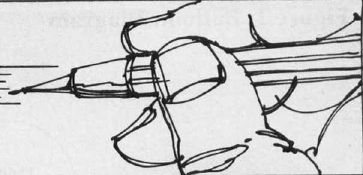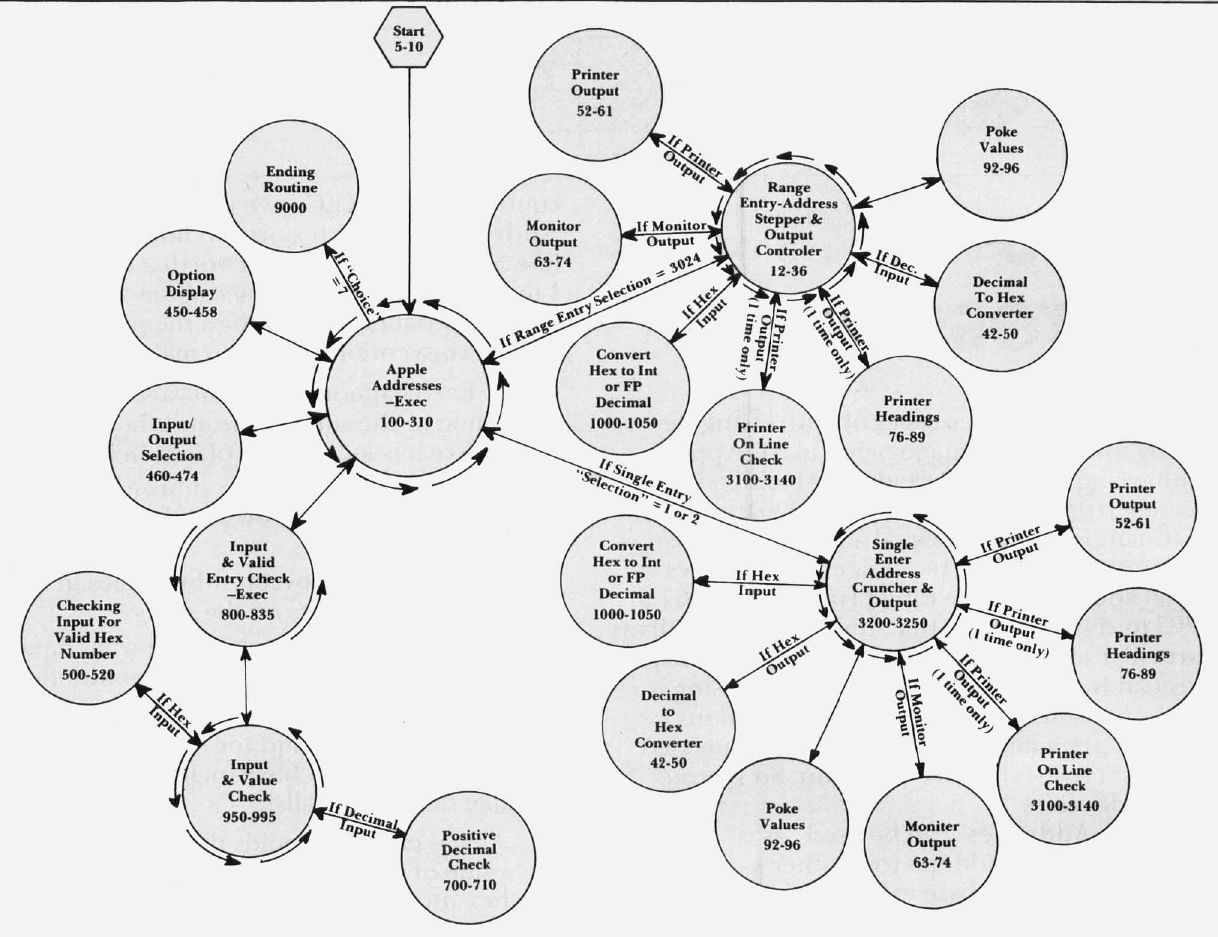`COMPUTE! ISSUE 20 / JANUARY 1982 / PAGE 83`

 THE apple® GAZETTEApple Addresses Bill Grimm Mountain View, CA The Apple II uses three types of addressing depending upon the language being used. Apple's machine language uses hexadecimal addresses in the range from \$0000 to \$FFFF. Its Floating Point BASIC language uses decimal addresses in the range from 0 to 65535. Its Integer BASIC uses decimal addresses in the range from 0 to 32767 to -32767 to -1. This means that, if you want to address a particular memory location, you must choose the correct address for the language you are using. Since I program in all three languages and my references are a mixture from all three, I needed an address cross-reference program. So I wrote "Apple Addresses." "Apple Addresses" can be used "as is" to convert one language's address to another's, and to give the high and low byte values which need to be POKEd into a BASIC program to store that address. Alternatively, you could extract the subroutines in Apple Addresses which convert between hex and decimal numbers and insert them in your own program. See the last paragraph of this article for more details. The program begins by asking the user which of the six possible conversions he would like to make. This is followed by a request to select the way the results of the conversions are to be displayed. There are four possible displays: single conversions displayed on the monitor one at a time. single conversions printed out on a Silentype printer* one at a time. a range of conversions displayed on the monitor. a range of conversions printed out on a Silentype printer*. *With slight program modifications other printers could be used. Subroutines "Apple Addresses" makes extensive use of subroutines. This helps in organizing the program as well as making it shorter and easier to debug. The controlling or EXECutive routine is called Apple Addresses—Exec. It starts on line 100 and goes to line 310. Since a picture is worth a thousand words, I made what I call a balloon diagram (Figure 1) to show how data flows through the program. These are the conventions I used to make the diagram; Each balloon represents a subroutine. The name of the subroutine and the line numbers where it is located are placed in the balloon. Data flows through a subroutine in the direction of the arrows on the outside of the balloon. Data flows between subroutines in the direction of the arrows on the strings. If conditions are placed on what data flows through a subroutine, these conditions are written in along the strings. As an additional aid for understanding how the program works I have included the following variable descriptions list: A()— each A(I) holds the decimal equivalent value of the Ith hexadecimal numeral in the hex number being created from a decimal number—appropriate numbers are then added to convert these to ASCII codes. A\$()—holds the characters represented by the ASCII codes in A(). CHOICE—holds the number of the conversion chosen—see lines 120 to 178. DVL—holds the decimal value of the number being converted—may be either FP or INT decimal. DVL\$—is the string equivalent of DVL and is used in the output routines. FLAG—if FLAG = 1 then an invalid number was entered and the program returns to get a new number. FRST—holds the FP BASIC address equivalent of the lowest address in the selected range. FRST—holds the FP BASIC address equivalent of the lowest address in the selected range. FRST\$—holds the smallest address chosen—this address is then processed and stored in FRST. HVL\$—holds the hex number selected or the hex number resulting from the conversion—if no hex numbers are involved then it holds the converted decimal number. LST—holds the FP BASIC address equivalent of the largest address in the selected range. Figure 1: Balloon DiagramLST\$—holds the largest address chosen—this address is then processed and stored in LST. N—holds the decimal equivalent of each hex numeral in a hex number being converted to a decimal number. PHI%—holds the number that would be POKEd into the high byte when placing the address into memory. PLO%—holds the number that would be POKEd into the low byte when placing the address into memory. POK—holds the address from which PLO% and PHI% are derived. SELECT—holds the type of output selected—see lines 462 to 470. STP—holds the positive decimal stepping interval chosen. STP\$—holds the stepping interval chosen which is later changed and stored in STP. TB—the horizontal tab value desired. TN—holds the intermediate numbers of the decimal address that is being converted into a hex address. VTB—used to control the vertical tabbing of the monitor output. Some Suggestions I have found that the easiest way to debug a program while I am entering it is to first type in the EXEC program. Then, if I place return statements at all the branching locations, I can check the EXEC for bugs. Once the EXEC is free of bugs, I add one subroutine at a time in the order that the EXEC uses them, checking for bugs as I go. If you have a need for subroutines which convert numbers from hex to decimal or from decimal to hex, two subroutines in this program may be of help. The first is called "decimal to hex converter" (lines 42 to 50). The input to this routine is TN which must hold a positive decimal number <65536. The output is HVL\$ which holds the hex equivalent to the number in TN. The second is called "convert hex to INT or FP decimal" (lines 1000 to 1050). The input to this routine is HVL\$ which must hold a hex number < = \$FFFF and choice. If choice = 1 then you get the positive decimal equivalent. Otherwise you get Int BASIC's equivalent. The output is a decimal number in DVL.